## Screenshots

•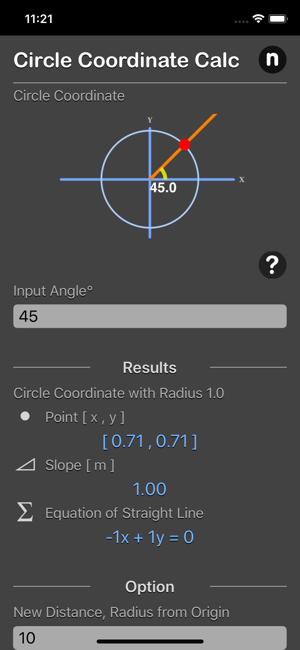•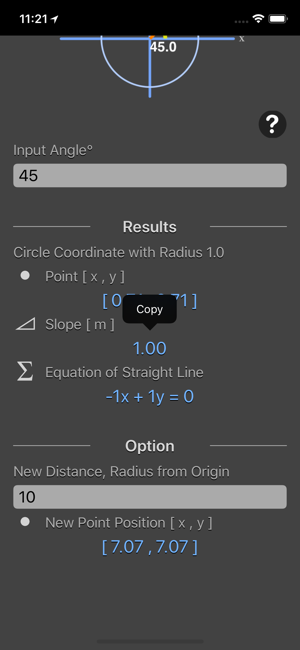•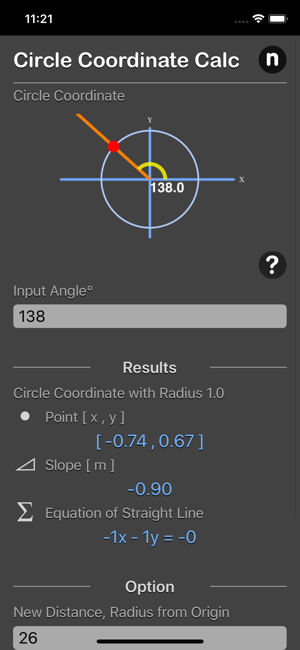•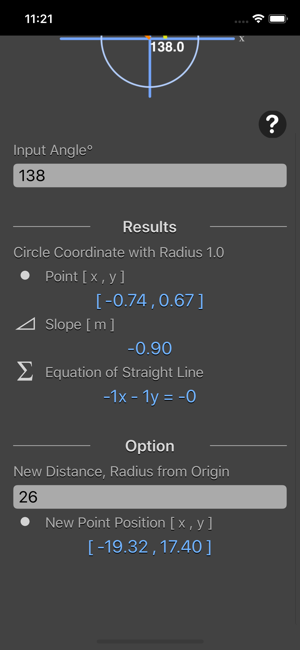•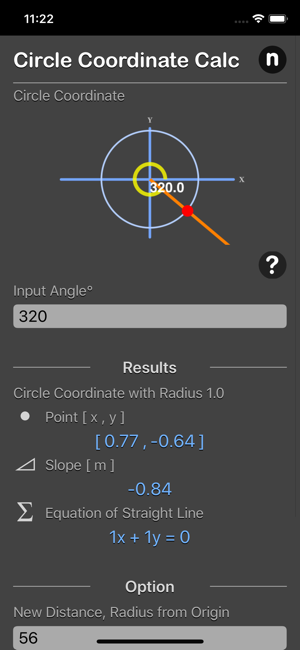•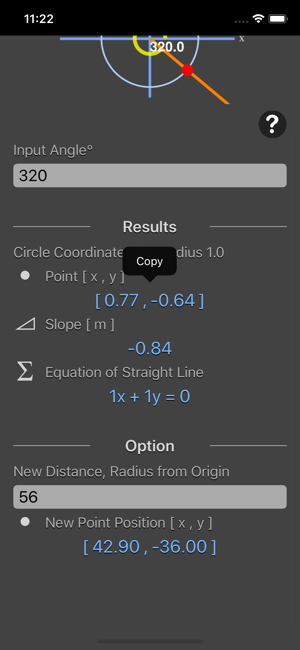## Description

Unit Circle Coordinate Calculator calculates the sine, cosine (x , y) values of any given angle.

Features:
- Calculates the sine, cosine (x , y) values of any given angle.
- Calculates the slope (m) for the line
- Output the formula for the line
- Output the x coordinate and y coordinate by given new distance from origin
- Result are instant and copy-able

Unit circle is a circle which is centered at origin and which has the radius of 1 unit. It is represented by the equation:
x^2 + y^2 = 1.

The values of x and y are given by trigonometric functions as:
x = sin θ
y = cos θ

So the value of sin θ and cos θ should such that the sum of their squares should be equal to 1.

*This is a universal app that work for both iPhone and iPad.

Thanks for your support and do visit nitrio.com for more apps for your iOS devices.

## Information

Provider
Tan Chia Ling
Size
16.2 MB
Category
Utilities
Compatibility

Requires iOS 8.0 or later. Compatible with iPhone, iPad and iPod touch.

Languages

English

Age Rating
4+
Price
₹ 249

## Supports

•